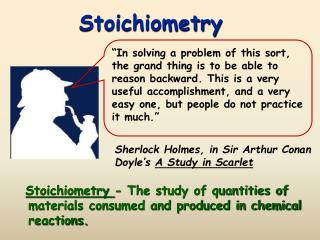DownloadDownload PresentationStoichiometry

# Stoichiometry

Télécharger la présentation## Stoichiometry

- - - - - - - - - - - - - - - - - - - - - - - - - - - E N D - - - - - - - - - - - - - - - - - - - - - - - - - - -
##### Presentation Transcript

1. Stoichiometry “In solving a problem of this sort, the grand thing is to be able to reason backward. This is a very useful accomplishment, and a very easy one, but people do not practice it much.” Stoichiometry- The study of quantities of materials consumed and produced in chemical reactions. Sherlock Holmes, in Sir Arthur Conan Doyle’s A Study in Scarlet

2. CA Standards

3. Calculating Masses of Reactants and Products • Balance the equation. • Convert mass or volume to moles, if necessary. • Set up mole ratios. • Use mole ratios to calculate moles of desired substituent. • Convert moles to mass or volume, if necessary.

4. Working a Stoichiometry Problem 6.50 grams of aluminum reacts with an excess of oxygen. How many grams of aluminum oxide are formed. 1. Identify reactants and products and write the balanced equation. 4 Al + 3 O2 2 Al2O3 a. Every reaction needs a yield sign! b. What are the reactants? c. What are the products? d. What are the balanced coefficients?

5. Working a Stoichiometry Problem 6.50 grams of aluminum reacts with an excess of oxygen. How many grams of aluminum oxide are formed? 4 Al + 3O2 2Al2O3 6.50 x 2 x 101.96÷ 26.98 ÷ 4 = 12.3 g Al2O3 6.50 g Al 1 mol Al 2 mol Al2O3 101.96 g Al2O3 = ? g Al2O3 4 mol Al 1 mol Al2O3 26.98 g Al

6. Gas Stoichiometry #1 If reactants and products are at the same conditions of temperature and pressure, then mole ratios of gases are also volume ratios. 3 H2(g) + N2(g)  2NH3(g) 3moles H2 +1mole N2 2moles NH3 3liters H2 + 1liter N2 2liters NH3

7. Gas Stoichiometry #2 How many liters of ammonia can be produced when 12 liters of hydrogen react with an excess of nitrogen? 3 H2(g) + N2(g)  2NH3(g) 12 L H2 2 L NH3 = L NH3 8.0 3 L H2

8. Gas Stoichiometry #3 How many liters of oxygen gas, at STP, can be collected from the complete decomposition of 50.0 grams of potassium chlorate? 2 KClO3(s)  2 KCl(s) + 3 O2(g) 50.0 g KClO3 1 mol KClO3 3 mol O2 22.4 L O2 122.55 g KClO3 2 mol KClO3 1 mol O2 = 13.7 L O2

9. Gas Stoichiometry #4 How many moles of oxygen gas, at 37.0C and 0.930 atmospheres, can be collected from the complete decomposition of 50.0 grams of potassium chlorate? 2KClO3(s)  2KCl(s) + 3O2(g) 50.0 g KClO3 1 mol KClO3 3mol O2 0.612 = mol O2 122.55 g KClO3 2mol KClO3